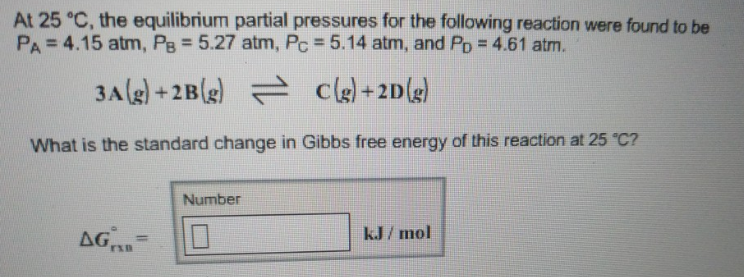# Problem: At 25°C, the equilibrium partial pressures for the following reaction were found to be PA = 4.15 atm, PB = 5.27 atm, PC = 5.14 atm, and PD = 4.61 atm. 3A (g) + 2B (g) ⇌ C (g) + 2D (g) What is the standard change in Gibbs free energy of this reaction at 25°C?

###### FREE Expert Solution
93% (469 ratings)###### Problem Details

At 25°C, the equilibrium partial pressures for the following reaction were found to be PA = 4.15 atm, PB = 5.27 atm, PC = 5.14 atm, and PD = 4.61 atm.

3A (g) + 2B (g) ⇌ C (g) + 2D (g)

What is the standard change in Gibbs free energy of this reaction at 25°C?